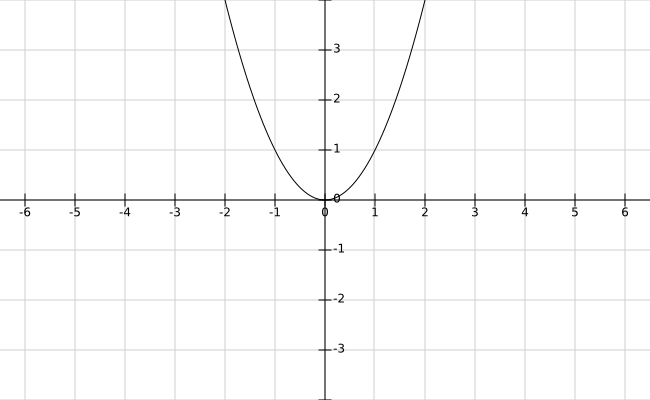# Square Function – Graph, Definition, Domain and Range

Here you will learn what is square function with definition, graph, domain and range.

Let’s begin –

## Square Function

The function that associates a real number x to its square i.e. $$x^2$$ is called square function. Since $$x^2$$ is defined for all x $$\in$$ R. So, we defined the square function as follows :

Definition : The function f : R $$\rightarrow$$ R defined by f(x) = $$x^2$$ is called the square function.

## Square Function Graph

The graph of f(x) = $$x^2$$ is :## Domain and Range :

Clearly, the domain of the square function is R and its range is set of all non-negative real numbers i.e. [$$0, \infty$$).

Domain : R

Range : [$$0, \infty$$)